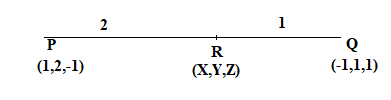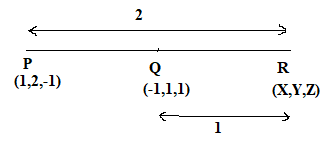Courses
Courses for Kids
Free study material
Free LIVE classes
MoreLIVE
Join Vedantu’s FREE Mastercalss

# Find the position vector of a point R which divides the line joining two points P and Q whose position vectors are $\mathop {\text{i}}\limits^ \wedge + 2\mathop {\text{j}}\limits^ \wedge - \mathop {\text{k}}\limits^ \wedge$ and $- \mathop {\text{i}}\limits^ \wedge + \mathop {\text{j}}\limits^ \wedge + \mathop {\text{k}}\limits^ \wedge$ respectively in the ratio 2:1(I) Internally (II) ExternallyVerified
358.8k+ views
Hint: This is a question based on the section formula in 3D vector. The coordinates of two points P and Q in 3D are given and a line is formed by joining these points and we have to divide this line in 2:1 ratio. So, we will use section formula to find the coordinate of point R.

In the question, it is given that
Coordinates of point P is $\mathop {\text{i}}\limits^ \wedge + 2\mathop {\text{j}}\limits^ \wedge - \mathop {\text{k}}\limits^ \wedge$ .
Coordinates of point Q is $- \mathop {\text{i}}\limits^ \wedge + \mathop {\text{j}}\limits^ \wedge + \mathop {\text{k}}\limits^ \wedge$ .
(I)
The figure for case when R divides PQ internally is:We have assumed that the coordinate of point R is (X,Y,Z).
To find the coordinate of point R, we will use the section formula.
According to section formula, the coordinates of point R for the above case is given by:
${\text{X = }}\dfrac{{{\text{m}}{{\text{x}}_2} + {\text{n}}{{\text{x}}_1}}}{{{\text{m + n}}}} \\ {\text{Y = }}\dfrac{{{\text{m}}{{\text{y}}_2} + {\text{n}}{{\text{y}}_1}}}{{{\text{m + n}}}} \\ {\text{Z = }}\dfrac{{{\text{m}}{{\text{z}}_2} + {\text{n}}{{\text{z}}_1}}}{{{\text{m + n}}}} \\$

From the given question, we can write:
${{\text{x}}_2} = - 1,{{\text{x}}_1} = 1 \\ {{\text{y}}_2} = 1,{{\text{y}}_1} = 2 \\ {{\text{z}}_2} = 1,{{\text{z}}_1} = - 1 \\ {\text{and }}\dfrac{{{\text{PR}}}}{{{\text{RQ}}}} = \dfrac{{\text{m}}}{{\text{n}}} = \dfrac{2}{1}. \\$
Now, putting the above values in the section formula, we get:
${\text{X = }}\dfrac{{{\text{(2}} \times {\text{ - 1)}} + (1 \times 1)}}{{2 + 1}} = \dfrac{{ - 2 + 1}}{3} = \dfrac{{ - 1}}{3} \\ {\text{Y = }}\dfrac{{{\text{(2}} \times 1) + (1 \times 2)}}{{2 + 1}} = \dfrac{4}{3} \\ {\text{Z = }}\dfrac{{{\text{(2}} \times 1) + (1 \times - 1)}}{{2 + 1}} = \dfrac{{2 - 1}}{3} = \dfrac{1}{3} \\$
So, coordinates of point R is $\left( {\dfrac{{ - 1}}{3},\dfrac{4}{3},\dfrac{1}{3}} \right)$ for the case of internal division.

(II)
In this case it is given that Point R divides PQ externally.
The figure for this case is:Section formula for external division is given by:
${\text{X = }}\dfrac{{{\text{m}}{{\text{x}}_2}{\text{ - n}}{{\text{x}}_1}}}{{{\text{m + n}}}} \\ {\text{Y = }}\dfrac{{{\text{m}}{{\text{y}}_2}{\text{ - n}}{{\text{y}}_1}}}{{{\text{m + n}}}} \\ {\text{Z = }}\dfrac{{{\text{m}}{{\text{z}}_2}{\text{ - n}}{{\text{z}}_1}}}{{{\text{m + n}}}} \\$

Now, putting the above values in the section formula, we get:
${\text{X = }}\dfrac{{{\text{(2}} \times {\text{ - 1) - }}(1 \times 1)}}{{2 + 1}} = \dfrac{{ - 2 - 1}}{3} = \dfrac{{ - 3}}{3} = - 1 \\ {\text{Y = }}\dfrac{{{\text{(2}} \times 1) - (1 \times 2)}}{{2 + 1}} = 0 \\ {\text{Z = }}\dfrac{{{\text{(2}} \times 1) - (1 \times - 1)}}{{2 + 1}} = \dfrac{{2 + 1}}{3} = \dfrac{3}{3} = 1 \\$
So, coordinates of point R is $\left( { - 1,0,1} \right)$ for the case of external division.
Note: In this type of question, where 3D coordinates are given you should remember the section formula for 3D vector for both the cases i.e. external division and internal division. You should make a clear diagram for both the cases such that all the values are clearly visible.

Last updated date: 16th Sep 2023
Total views: 358.8k
Views today: 4.58k